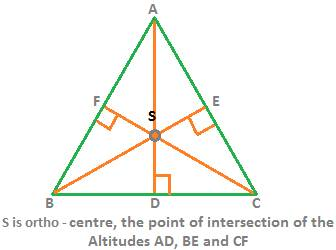## Various Types of Triangles

For a recap of concepts in triangles, click Triangles and Parts of triangles.

Lets move on with this lesson on Various Types of Triangles

Triangles are classified on two factors. They are

• Sides and
• Angles

The three types of triangles classified under sides or arms of a triangle are:

1. Scalene triangle:

A triangle in which all of the three sides are of different lengths.

2. Isosceles triangle:

A triangle in which any two sides have equal lengths.

In the above figure,  is isosceles. Because the two sides AB and AC are equal in length.

IMPORTANT RULE:

If two sides are of same length, then the angles opposite them are of same measure too.

Since AB = AC, therefore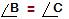A triangle in which all three sides are of equal lengths is equilateral triangle.

From angle sum property in a triangle, we know thatIn equilateral triangle, since,Therefore, each ofis 600

Acute Angled Triangle

A triangle in which every angle is acute, i.e. less than 900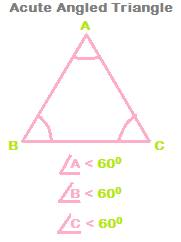Right Angled Triangle

A triangle in which one angle is exactly a right angle, i.e. 90<sup>0</sup>.

The right angle is denoted by a small square symbol at the vertex where it is formed.

The two sides which contain the right angle are called the legs of a right angled triangle.

The side opposite to the right angle is called Hypotenuse.

Hypotenuse is the longest side in a right angled triangle.

Two types of right angled triangle (R.A.T.) are very useful. They are:

• 300- 600-900 (R.A.T.) and
• 450 - 450 - 900 (R.A.T.)

Consider first the 300- 600-900 right angled triangle.

Let the length of the side opposite 300 be x, then, the length of the side opposite 600 will be √3 × x, and the length of the side opposite 900 is 2x.

The following figure will summarize the above properties of 300- 600-900 (R.A.T.) succinctly: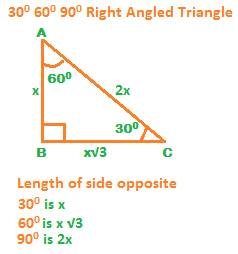• The other very useful type is the 450 - 450 - 900 right angled triangle.

Since two angles are equal, therefore opposite them the sides are of equal lengths.

Let the length of each side opposite the two 450 be x, then the length of the side opposite 900 will be √2 × x.

The following figure will clearly illustrate this case

The following table will encapsulate the above points

: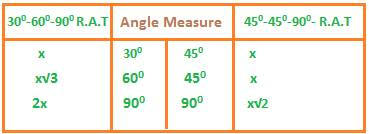Obtuse Angled Triangle:

A triangle in which one angle is obtuse (> 900) is called an obtuse angled triangle.

The side opposite the obtuse angle is the longest side in the triangle.

In the following obtuse angled triangle ABC, angle at C is obtuse and the side AB opposite it is the longest side.

The sum of the two angles other than the obtuse angle in an obtuse angled triangle is less than 900.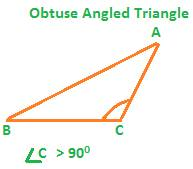Centroid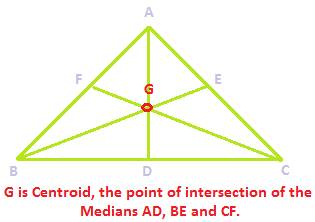Orthocentre